## Saturday, 24 December 2011

### Mathematical Induction and its consequences -- Finale

P.S. In before this starts. I decided to insert the method of induction into my summarizing work, Basic Albegraic Skills.

"Numbers" in history have different meanings. In the past there'are only natrual numbers by counting, next there was negative numbers due to the concept of debt. Rational numbers seems to fill up the real continum but Pythagoreans has found irrational numbers. Finally the concept of real number continum is developed by Cantor and complex numbers appeared as powerful calculation tool.

Mathematical induction, processing natrual numbers, seems too "old fashioned" because there're far many problems concerning somewhere beyond natrual numbers, and we need different approaches. If MI can be extended over the reals, it's just fantastic. We will illustrate this by two examples: function equation and bionomial theorem over the reals. Before that, we will look at more different MI problems on A-level exam past papers.

Theorem. (Generalization of M.I.)
If (a) P(a) and P(b) is true implies that P(a+b), P(|a-b|) is true,
b) Exist least r that P(r) is true,
then P(k) is true is equivalent to k is a multiple of r.

6. N, Z, Q, R --- extented conclusion derived from MI

Example 16. f(x) is a everywhere continuous and differentiable function satisfying:
1)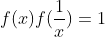for non-zero x;
2) Additive, i.e.,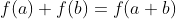;
3) f(1)=1.
Show that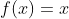.

Step 1: N
For the proprosition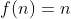: assume n=k is true, and for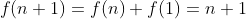is also true.

Step 2: Z, therefore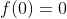.
Next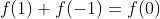, therefore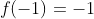.
Now set the proposition "f(-n)=-n" and finish the induction.

Another approach is that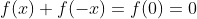, therefore f is even and it's true over integers.

Step 3: Q
We use a double MI:
Theorem. The proposition P(a,b) is true for all a,b if:
1) P(1,1) is true.
2) If P(a,b) is true, then P(a,b+1) is true.
3) If P(a,b) is true, then P(a+1,b) is true.
This is quite powerful for rational numbers.
Here we use another approach of double MI that "For every b, P(a,b) implies P(a+1,b) is true".
For any given n,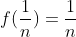by property 1, and do induction so that "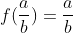for every b. The method is similar as step 1 and 2. This is true for all rational numbers.

Step 4: R
We know that irrational numbers can always be traced by a series of rational numbers.
Lemma. Every irrational numbers can be written in forms of sum of rational numbers.
Then if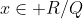and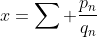, then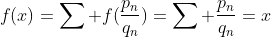.

P.S. Yet there's a simple method to do this question. Since the function is additive, we can show that the function has a constant slope, i.e., y' = m, so y = mx+c. Considering f(0)=0 and f(1)=1, we have f(x)=x.
Example 17. Extension of the binomial theorem.

Lemma. Vandermonde's Theorem: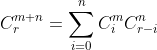The proof is trivial when you expand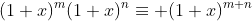and compare the coefficients of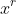.

Now consider the function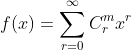where f converges when |x| < 1. Now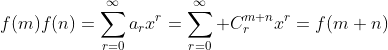(This can be done by direct expanding.)

Now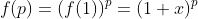so that it's also valid for real p.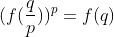, so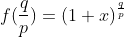in which the positive root is taken due to positive sum is given.

For negative numbers, we have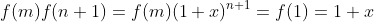, then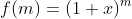.

For real numbers, we know that f is everywhere continous and differentiable in the interval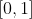, therefore we can also put a irrational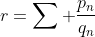that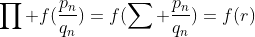.

By comparing the coefficients we have the binomial coefficients over real numbers: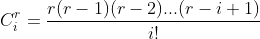and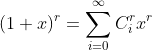. When r is integer,for r < n so that the sum is finite. These two results are quite powerful and the following problems can be solved. Exercise.
1) Show that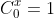for all real x.
2) Show that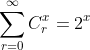is valid over real x by:
a) binomial substitution
b) the NZQR approach.
c) Compute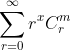where m and x is a real number.
3) Show the following identities:
a)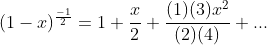b)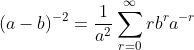where. Is it valid when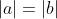?
4) (2004 Pure I 5 modified) For real x, |a| < 1, show that: a),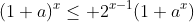, hence show that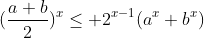Read the rest of this passage:

Part I
Part II
Part III

## Thursday, 22 December 2011

### Mathematical induction and its consequences - Part 3

In part 3 here we try to provide more examples and to conclude methods of M.I. So that in the Finale we could apply M.I. to wider cases.

5. Matrix and more examples

Example 12 (HKALE Pure 93 I 10) Let M be the set of 3x3 real matrices. For $A,B\in M$, a relation ~ is set if there exist a non-singular $P\in M$ so that $A=PBP^{-1}$, then $A-B$.

a) Show that ~ is an equivalence relation.
b) Show if $A\sim B$ then $A^n\sim B^n$

Not a really "MI" question but this is a rare question involving MI because proves on matrices rarely depends on integers and their proves would be extremely difficult.

Definition. A equivalence relation A~B is set if and only if:
1) A~A (Reflxivity)
2) A~B implies B~A (symmetry)
3) A~B, B~C implies A~C (Transitivity).

Example 13. Defind a~b if $a-b=c\in Q$, this ia an equivalence relation because:
1) $x-x=0\in Q$, therefore $x\sim x$.
2) $a-b=c\in Q$ implies $b-a=-c\in Q$ so it's symmetrical.
3) $a-b,b-c\in Q$ implies $a-c\in Q$ since rational number performs closure in addition.

Now back to the original problem:
1) $A=IAI^-1$, therefore A~A.
2) $A=PBP^{-1}$, then $B=(P^{-1})A(P^{-1})^{-1}$, so it's symmetrical.
3) If $A=PBP^{-1}$ where PQ is non-singular by determinants. For part (b) we have $A^n=(PBP^{-1})^n=PB^nP^{-1}$ so that $A^n\sim B^n$. How can we do this through MI?

A~B is the case n=1 which is given. Assume $A^n\sim B^n$ by $A^n=PB^nP^{-1}$, then $A^{n+1}=(PB^nP^{-1})(PBP^{-1})=PB^{n+1}P^{-1}$ and the same result is given, though this is quite stupid.

Readers can try to prove the following:
1) If C~0, then C is zero matrix.
2) AB ~ BA may not be true.

<i>Example 14. (HKALE Pure 1992 I 5</i> Given that $u_1=0$, $u_{n+1}=2n-u_n$, show that $u_n=n+\frac{-1+(-1)^n}{2}$.

Approach 1: induction through odd and even separately.
We have $u_{n+2}=2+u_n$ so that it's true by summing up from the first term ($u_1$ for odd terms and $u_2$ for even terms).

Approach 2: induction through all integers.
Assume $u_n=n+\frac{-1+(-1)^n}{2}$, then $u_{n+1}=2n-u_n=n-\frac{-1+(-1)^n}{2}=(n+1)+\frac{-1+(-1)^{n+1}}{2}$ so that the induction is complete.

<i>Example 15. (HKALE Puer 1989 I 11a)</i> Prove the existance and uniqueness of $a_n,b_n$ so that $(1+\sqrt{2})^n=a_n+b_n\sqrt{2}$ and also:
i) $a_n\equiv 1\mod 2$ for all n.
ii) $b_n\equiv 1\mod 2$ for odd n.

Existance is trivial as the multiplication of $Z[\sqrt{2}]$ (lattice point in forms of $a+b\sqrt{2}$ that a,b are integers) performs closure, and there's at least one solution $(a_n,b_n)$ for every n. This is unique because $\sqrt{2}$ is irrational so that $a\sqrt{2}=b$ don't give rational solution.

For n=1, a=b=1.
Part I: Assume $a_n$ is odd for n = k. For n = k+1,
$(a_n+b_n\sqrt{2})(1+\sqrt{2})=(a_n+2b_n)+(a_n+b_n)\sqrt{2}=a_{n+1}+b_{n+1}\sqrt{2}$ so that $a_{n+1}=a_n+2b_n\equiv 1\mod 2$.
Part II:Notice that $(1+\sqrt{2})^2=5+2\sqrt{2}$, tweet the proposition that for $(1+\sqrt{2})(5+2\sqrt{2})^{n-1}=c_n+d_n\sqrt{2}$, $d_n\equiv 1\mod 2$. Assume that n = k is true, then for n = k+1,
$(c_n+d_n\sqrt{2})(5+2\sqrt{2})=(5c_n+4d_n)+(5d_n+2c_n)\sqrt{2}$ so that $d_{n+1}$ is also odd.

To end this one we would introduce a special case of M.I.
<i>Theorem. (M.I. from the middle)</i> The proposition is true for all n if:
a) P(k) is true, k is not necessarily 1.
b) Assuming P(x) is true, then P(x+1) and P(x-1) is true.
If P(x-1) is not necessarily true, then there exist $n_0\leq k$ that for all $n\geq n_0$, P(n) is true.

Somehow we don't have many elemental case for this because most proofs involving M.I., is true from n=1. Such bounding occurs more frequently in inequality and bounding case which could be much difficult. There's a modified question at the bottom (Q1) concerning this M.I. method.

To conclude, the followings are usual techniques for M.I.:
1) Expressing the case n = k+1 in terms of n = k, including their difference.
2) Seperate odd and even case especially in sequence.
3) Artificially make up expression, like $\frac{(n+2)(n+3)}{2}=\frac{((n+1)+1)((n+1)+2)}{2}$ as to show the case n = k+1
4) For M.I. in inequality, usually they behaves monotonically as power increases, so observing pattern is important.

Problems from HK A-Level Examinations:
1) (HKALE 1993 Pure I 2 modified)
$u_8=47,u_{11}=199$ and $u_{n+2}=u_{n+1}+u_n$ for all natrual n. Show that $u_n=\alpha^n+\beta^n$ where $\alpha, \beta$ are roots of $x^2-x-1=0$ by:
a) Finding $u_1,u_2$ and induction through natrual n.
b) By M.I. from the middle.

2) (HKALE 1996 Pure II 7b)
Show that $\sum_{r=0}^n \frac{x^r}{r!}+\frac{ex^{n+1}}{(n+1)!} > e^x > \sum_{r=0}^n \frac{x^r}{r!}$, hence show that $\sum_{r=0}^n \frac{x^r}{r!}$ tends to $e^x$ the constant.

3) (HKALE 1991 Pure I 11)
a) Prove the existance and uniqueness that $(\sqrt{3}+\sqrt{2})^{2n}=p_n+q_n\sqrt{6}$ for natrual $n,p_n,q_n$. Also show that $(\sqrt{3}-\sqrt{2})^{2n}=p_n-q_n\sqrt{6}$. Hence deduce that $2p_n-1$ < $(\sqrt{3}+\sqrt{2})^{2n}$ < $2p_n$.
b) Show that the followings are multiples of 10:
i) $2^{5n}-2^n$
ii) $3^{4n}-1$
iii) $2p_{2n}-(2^{3n+1})(3^n)$.
c) By (a),(b) or otherwise, find the unit digit of $[(\sqrt{3}+\sqrt{2})^{100}]$ when it's expressed in decimal form. Note that [] is the integer (floor) function.
Read the rest of this passage:
Part I
Part II
Part IV

## Friday, 16 December 2011

### Mathematical induction and its consequences Part 2

3. M.I. VS mod
In most case, M.I. would not be the most preferred choice to prove the divisibility of an expression because we have another powerful tool: modolar arithmetic.

I would not put too much technical formulae about mod because it could be very difficult.

Definition 1. If $a=b+n$ where n is a positive integer larger than 1, then we say $a\equiv b\mod n$.

Corollary 1. If a is divisible by b, then $a\equiv 0\mod b$.

Corollary 2. There's a unique, least positive integer so that for every a we have b so that $a\equiv b\mod n$, we can say b is the principal value of a mod n, or the remainder when a is divided by n.

Corollary 3. $a+bm\equiv a\mod n$, then by binomial theorem, $(a+bn)^k\equiv a^k \mod n$.

Proof: exercise.

By such method we can now proof all previous examples.

Example 7. (Example 3 - 4 revisited) $6^n\equiv 1\mod 5$ and $6^n(5n-1)\equiv -1\mod 5$
The first one is trivial because $6^n=(1+5)^n\equiv -1\mod 25$.
The second statement can't be proved by diredctly proved in mod 25. Instead we show that $\frac{6^n(5n-1)+1}{5}\equiv 0\mod5$.
$\frac{6^n(5n-1)+1}{5}=n6^n+(1+6+6^2+...+6^{n-1})\equiv n-n=0\mod 5$.

For example 6, we know that $7\pm \sqrt{13}$ are the roots of $x^2-14x+36=0$, by binomial theorem, $(7+\sqrt{13})^n+(7-\sqrt{13})^n=2(C^n_07^n+C^n_27^{n-2}(13)+...)$, but at this level we can't find the bridge to show the divisibility through mod, but we can actually prove this by M.I. through this formula.

Lemma 1. (Extension of M.I.) The proposition is true for all natrual number n if:
a) The proposition is true for n = 1,2,3...,i
b) Assuming n = k is correct, then n = i + k is correct.

From (b), for every $a\equiv b\mod n$ where a is smaller than b, if n = a is true, then n = b is true, eventually it's true for all natrual number n.

By dividing the case into odd n and even n and complete M.I. separately, we can prove the statement as well.

4. Sequence and inequality

Example 8. (HKALE 1994 Pure I2) If $\sum a_i=(\frac{1+a_n}{2})^2$, prove that $a_n=2n-1$ if all terms are positive numbers.

For sequence, we usually prove the n=k+1 part by comparing their difference.

Define $s_n=\sum^{n}_{i=1}a_i=\frac{1}{4}(1+a_n)^2$.
Now $a_{n+1}=s_{n+1}-s_n$. Assuming that $a_n=2n-1$, then $s_n=\frac{1}{4}(1+2n+1)^2=n^2+2n+1$.

Now $a_{n+1}=\frac{1}{4}(1+a_{n+1})^2-(n+1)^2$
$\frac{1}{4}(1-a_{n+1})^2=(n+1)^2$,
$a_{n+1}=2n+1$, where all negative terms are rejected.

Apart from some trivial geometric sequences, some sequences can also be transferred into something like geometric sequences, and we will introduce one of the sequence in form of $a_n=c+dr^n$.

Example 9. Find the general term of sequence $a_{n+1}=xa_n+y$.

Define $b_n=a_{n+1}-a_n$, then $b_{n+1}=a_{n+2}-a_{n+1}=(xa_{n+1}+y)-(xa_n+y)=x(a_{n+1}-a_n)=xb_{n-1}$, therefore $b_n=x^{n-1}(a_2-a_1)$ is a geometric sequence. Now $a_n=a_1+b_1+b_2+...+b_{n-1}=a_1+(a_2-a_1)(1+x+...+x^{n-2})$
$=a_1+(a_2-a_1)\frac{1-x^{n-1}}{1-x}$.

One of the stupid example is that $a_1=1$, $a_n=2a_{n-1}-1$, then $a_2=1$, $a_n=1+(1-1)\frac{1-2^{n-1}}{1-2}=1$, and therefore the sequence {1,1,1,1,...} is an A.S., G.S., as well as a recurrence sequence.

Exercise 4. It's given that the sum of a sequence is given by $S_n=4a_n+3n-4$, find its general term by
i) Guess and proved by M.I.
ii) Sequential analysis

Example 10. (HKALE 1995 Pure I6) For a non-negative integral integral sequence so that $n\leq \sum^{n}_{i=1}a_i^2\leq n+1+(-1)^n$ for all natrual number n. Prove that the sequence is identically 1.
This inequality is quite trivial.
Proof 1. $1\leq a_{n+1}^2\leq 3$ when we subtract case (n+1) from case n, since it's non-negative integer, $a_i=1$.
Proof 2. We use another lemma from MI.

Lemma 2. The proposition is true for all natrual n if:
1) n = 1 is true.
2) if n = 1,2,...,(k-1) is true, then n = k is true.

Assuming that $a_1=a_2=...=a_n=1$, then $n+1\leq n+a_{n+1}^2\leq n+2+(-11)^n\leq n+3$, the same result is given.

Exercise 5. Try to prove the above statement by separating n into odd and even case.

Example 11 (BAS ex. 9.4.8, p.84) For positive real so that $\prod (1+a_i)=2^n$, show that $\prod a_i \leq 1$.
Assuming n = k is true.
Now if one more number $a_{k+1}$ is added that $\prod (1+a_n)=2^{k+1}$ with $\sigma a_i$ greater than 1.

Then $a_{n+1}=(a_1a_2...a_n)^-1$ which is greater than 1, then $\prod (1+a_i)=2^n(1+a_{n+1})\neq 2^{n+1}$.

The above proof is incomplete and it would be nice for readers to finish the proof himself.

Read the rest of this passage:

Part I
Part III
Part IV

## Wednesday, 14 December 2011

### Mathematical induction and its consequences Part 1

Here we would concentrate in illustrating the case n = k + 1 assuming n = k is correct with n = 1 is correct.

1. Polynomials

Example. The most stupid M.I. in the world, showing that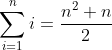.
In polynomial form M.I. is always easy: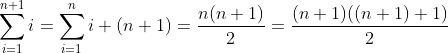so that the proof is complete.

Example 2.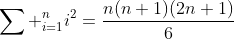Proof.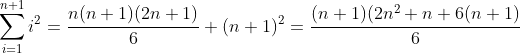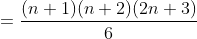which finishes the proof.

Exercise 1. Show that:
i)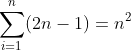, e.g.,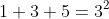ii)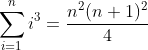In fact, there's no such a general form for sum of the n-th power of natrual number.

2. Divisibility and integral solution

Example 3.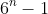is divisible by 5.
Let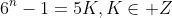, then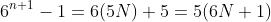.

In more complicated case, some of the grouping method may not work.

Example 4.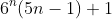is divisible by 25.
Let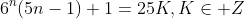, then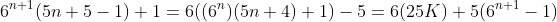where the last term is divisible by 25 by ex.3.

Example 5. (CSMO)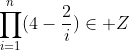for all positive integer n.
It seems to be very fresh to most M2 or even Pure student as you don't know the proposition to be set in order to prove the statement.Think inversely, the next product must be integer if the last one is a multiple, (and of course, an integer) of the next i, then the next term must be integer too.
Therefore the proposition is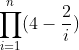is a multiple of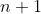.

Exercise 2. Show that
1)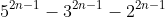is divisible by 15 by induction on n.
2) Finish the proof for ex. 5.

Challenge 1. It's given that the first 4 terms are 2,6,20,70,...
a) Give a general formula for the series with proof.
b) The series satisfies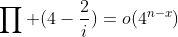, describe x.

The last example is from HKALE.

Example 6. (HKALE Pure 1998, I5) For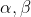as roots of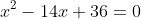, show that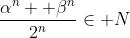for all natrual number n.

This is a 5-mark question and shouldn't be hard, or even fit perfectly for M2/Pure level, however we need another method of Mathematical Induction:

Theorem. Second principle of M.I. The proposition is true for all natrual n if:
1) n = 1,2,...i is true.
2) If n = j, (j+1), ... i+j is true, then n = i+j+1 is true.

in this case i = 2.
Assume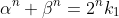and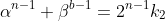.
By relationship between roots and equations, we have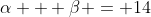,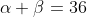.

Then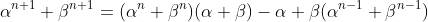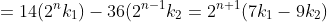The last term is trivially a positive integer.

Challenge 2. The recurrence relationship of the sequenceis shown. Find the general term of the sequence.

Exercise 3. Is it true that for x,y as roots of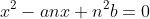satisfies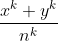is an integer? Prove your assertion. (a,b,n are natrual numbers.)

Read the rest of this passage:

Part II
Part III
Part IV